# Introduction

PKNCA considers two types of data grouping within data sets: the group and the interval. A group typically identifies a single subject given a single intervention type (a “treatment”) with a single analyte. An interval subsets a group by times within the group, and primary noncompartmental analysis (NCA) calculations are performed within an interval.

As a concrete example, consider the figure below shows the concentration-time profile of a study subject in a multiple-dose study. The group is all points in the figure, and the interval for the last day (144 to 168 hr) is the area with blue shading.

## Formula for concentration:
##  conc ~ time | treatment + ID
## With 1 subjects defined in the 'ID' column.
## Nominal time column is not specified.
##
## First 6 rows of concentration data:
##    study treatment ID time      conc   analyte exclude volume duration
##  Study 1     Trt 1  1    0 0.0000000 Analyte 1    <NA>     NA        0
##  Study 1     Trt 1  1    1 0.6140526 Analyte 1    <NA>     NA        0
##  Study 1     Trt 1  1    2 0.8100022 Analyte 1    <NA>     NA        0
##  Study 1     Trt 1  1    4 0.8425422 Analyte 1    <NA>     NA        0
##  Study 1     Trt 1  1    6 0.7771994 Analyte 1    <NA>     NA        0
##  Study 1     Trt 1  1    8 0.7052469 Analyte 1    <NA>     NA        0
# Plot the concentration-time data and the interval
ggplot(d_conc_multi, aes(x=time, y=conc)) +
geom_ribbon(data=d_conc$data, aes(ymax=conc, ymin=0), fill="skyblue") + geom_point() + geom_line() + scale_x_continuous(breaks=seq(0, 72, by=12)) + scale_y_continuous(limits=c(0, NA)) + labs(x="Time Since First Dose (hr)", y="Concentration\n(arbitrary units)")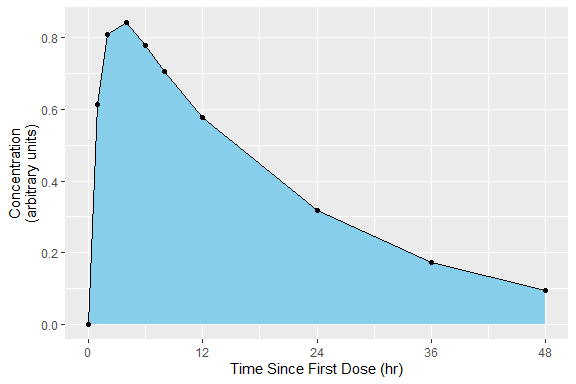intervals_manual <- data.frame( start=0, end=Inf, auclast=TRUE, aucinf.obs=TRUE ) print(intervals_manual) ## start end auclast aucinf.obs ## 1 0 Inf TRUE TRUE my.data <- PKNCAdata(d_conc, intervals=intervals_manual) ## Multiple Intervals More than one interval may be specified for the same subject or group of subjects by providing more than one row of interval specifications. In the figure below, the blue and green shaded regions indicate the first and second rows of the intervals, respectively. ## Formula for concentration: ## conc ~ time | treatment + ID ## With 1 subjects defined in the 'ID' column. ## Nominal time column is not specified. ## ## First 6 rows of concentration data: ## study treatment ID time conc analyte exclude volume duration ## Study 1 Trt 1 1 0 0.0000000 Analyte 1 <NA> NA 0 ## Study 1 Trt 1 1 1 0.6140526 Analyte 1 <NA> NA 0 ## Study 1 Trt 1 1 2 0.8100022 Analyte 1 <NA> NA 0 ## Study 1 Trt 1 1 4 0.8425422 Analyte 1 <NA> NA 0 ## Study 1 Trt 1 1 6 0.7771994 Analyte 1 <NA> NA 0 ## Study 1 Trt 1 1 8 0.7052469 Analyte 1 <NA> NA 0 # Plot the concentration-time data and the interval ggplot(d_conc_multi, aes(x=time, y=conc)) + geom_ribbon(data=d_conc_multi[d_conc_multi$time <= 24,],
aes(ymax=conc, ymin=0),
fill="skyblue") +
geom_ribbon(data=d_conc_multi[d_conc_multi$time >= 144,], aes(ymax=conc, ymin=0), fill="lightgreen") + geom_point() + geom_line() + scale_x_continuous(breaks=seq(0, 168, by=12)) + scale_y_continuous(limits=c(0, NA)) + labs(x="Time Since First Dose (hr)", y="Concentration\n(arbitrary units)")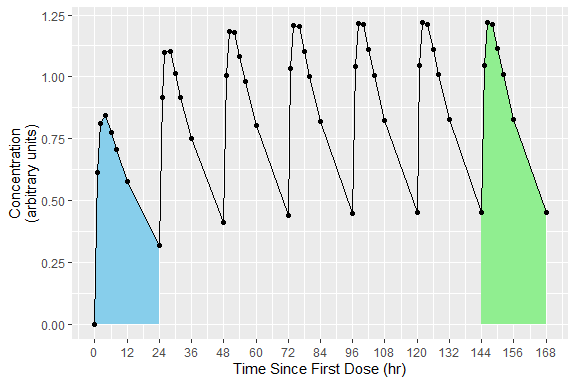intervals_manual <- data.frame( start=c(0, 144), end=c(24, 168), auclast=TRUE ) knitr::kable(intervals_manual) start end auclast 0 24 TRUE 144 168 TRUE my.data <- PKNCAdata(d_conc, intervals=intervals_manual) # Overlapping Intervals and Different Calculations by Interval In some scenarios, multiple intervals may be needed where some intervals overlap. There is no issue with an interval specification that has two rows with overlapping times; the rows are considered separately. In the example below, the 0-24 interval is shared between both the first and second (shaded blue-green). The example of overlapping intervals also illustrates that different calculations can be performed in different intervals. In this case, auclast is calculated in both intervals while aucinf.obs is only calculated in the 0-Inf interval. ## Formula for concentration: ## conc ~ time | treatment + ID ## With 1 subjects defined in the 'ID' column. ## Nominal time column is not specified. ## ## First 6 rows of concentration data: ## study treatment ID time conc analyte exclude volume duration ## Study 1 Trt 1 1 0 0.0000000 Analyte 1 <NA> NA 0 ## Study 1 Trt 1 1 1 0.6140526 Analyte 1 <NA> NA 0 ## Study 1 Trt 1 1 2 0.8100022 Analyte 1 <NA> NA 0 ## Study 1 Trt 1 1 4 0.8425422 Analyte 1 <NA> NA 0 ## Study 1 Trt 1 1 6 0.7771994 Analyte 1 <NA> NA 0 ## Study 1 Trt 1 1 8 0.7052469 Analyte 1 <NA> NA 0 # Use superposition to simulate multiple doses ggplot(d_conc$data, aes(x=time, y=conc)) +
geom_ribbon(data=d_conc$data, aes(ymax=conc, ymin=0), fill="lightgreen", alpha=0.5) + geom_ribbon(data=d_conc$data[d_conc$data$time <= 24,],
aes(ymax=conc, ymin=0),
fill="skyblue",
alpha=0.5) +
geom_point() + geom_line() +
scale_x_continuous(breaks=seq(0, 168, by=12)) +
scale_y_continuous(limits=c(0, NA)) +
labs(x="Time Since First Dose (hr)",
y="Concentration\n(arbitrary units)")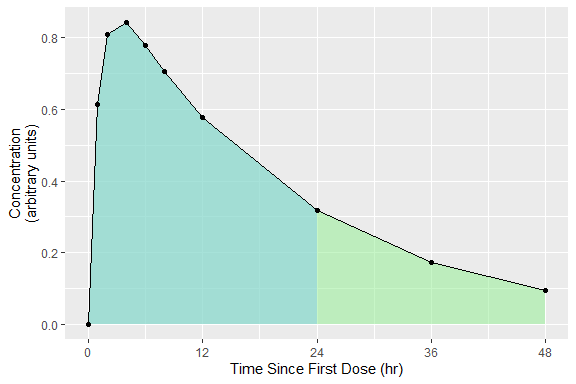intervals_manual <-
data.frame(
start=0,
end=c(24, Inf),
auclast=TRUE,
aucinf.obs=c(FALSE, TRUE)
)
knitr::kable(intervals_manual)
start end auclast aucinf.obs
0 24 TRUE FALSE
0 Inf TRUE TRUE
my.data <- PKNCAdata(d_conc, intervals=intervals_manual)

# Intervals with Duration

Some events have durations of times rather than instants in time associated with them. Two typical examples of duration data in NCA are intravenous infusions and urine or fecal sample collections. Inform PKNCA of durations with the duration argument to the PKNCAdose and PKNCAconc functions.

Durations data are selected based on both the beginning and ending of the duration existing within the interval.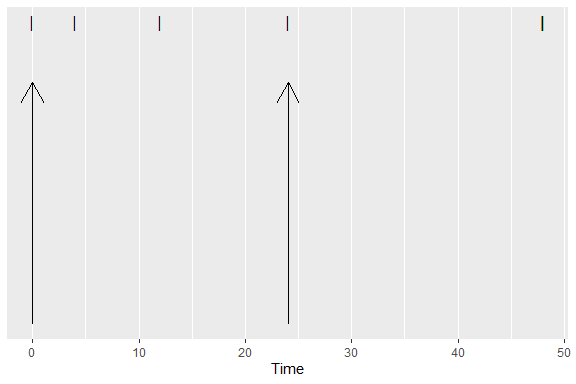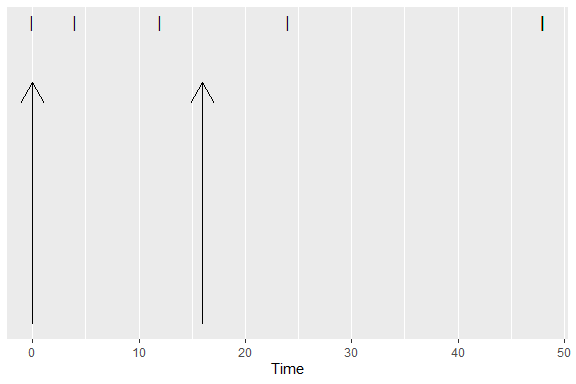# Parameters Available for Calculation in an Interval

The following parameters are available in an interval. For more information about the parameter, see the documentation for the function.

Parameter Name Parameter Description Function for Calculation
adj.r.squared The adjusted r^2 value of the half-life calculation See the parameter name half.life
ae The amount excreted (typically into urine or feces) pk.calc.ae
aucall The area under the concentration time curve from the beginning of the interval to the last concentration above the limit of quantification plus the triangle from that last concentration to 0 at the first concentration below the limit of quantification pk.calc.auc.all
aucall.dn Dose normalized aucall pk.calc.dn
aucinf.obs The area under the concentration time curve from the beginning of the interval to infinity with extrapolation to infinity from the observed Clast pk.calc.auc.inf.obs
aucinf.obs.dn Dose normalized aucinf.obs pk.calc.dn
aucinf.pred The area under the concentration time curve from the beginning of the interval to infinity with extrapolation to infinity from the predicted Clast pk.calc.auc.inf.pred
aucinf.pred.dn Dose normalized aucinf.pred pk.calc.dn
aucint.all The area under the concentration time curve in the interval extrapolating from Tlast to infinity with the triangle from Tlast to the next point and zero thereafter (matching AUCall) pk.calc.aucint.all
aucint.all.dose The area under the concentration time curve in the interval extrapolating from Tlast to infinity with the triangle from Tlast to the next point and zero thereafter (matching AUCall) pk.calc.aucint.all
aucint.inf.obs The area under the concentration time curve in the interval extrapolating from Tlast to infinity with zeros (matching AUClast) pk.calc.aucint.inf.obs
aucint.inf.obs.dose The area under the concentration time curve in the interval extrapolating from Tlast to infinity with zeros (matching AUClast) pk.calc.aucint.inf.obs
aucint.inf.pred The area under the concentration time curve in the interval extrapolating from Tlast to infinity with the triangle from Tlast to the next point and zero thereafter (matching AUCall) pk.calc.aucint.inf.pred
aucint.inf.pred.dose The area under the concentration time curve in the interval extrapolating from Tlast to infinity with the triangle from Tlast to the next point and zero thereafter (matching AUCall) pk.calc.aucint.inf.pred
aucint.last The area under the concentration time curve in the interval extrapolating from Tlast to infinity with zeros (matching AUClast) pk.calc.aucint.last
aucint.last.dose The area under the concentration time curve in the interval extrapolating from Tlast to infinity with zeros (matching AUClast) pk.calc.aucint.last
auclast The area under the concentration time curve from the beginning of the interval to the last concentration above the limit of quantification pk.calc.auc.last
auclast.dn Dose normalized auclast pk.calc.dn
aucpext.obs Percent of the AUCinf that is extrapolated after Tlast calculated from the observed Clast pk.calc.aucpext
aucpext.pred Percent of the AUCinf that is extrapolated after Tlast calculated from the predicted Clast pk.calc.aucpext
aumcall The area under the concentration time moment curve from the beginning of the interval to the last concentration above the limit of quantification plus the moment of the triangle from that last concentration to 0 at the first concentration below the limit of quantification pk.calc.aumc.all
aumcall.dn Dose normalized aumcall pk.calc.dn
aumcinf.obs The area under the concentration time moment curve from the beginning of the interval to infinity with extrapolation to infinity from the observed Clast pk.calc.aumc.inf.obs
aumcinf.obs.dn Dose normalized aumcinf.obs pk.calc.dn
aumcinf.pred The area under the concentration time moment curve from the beginning of the interval to infinity with extrapolation to infinity from the predicted Clast pk.calc.aumc.inf.pred
aumcinf.pred.dn Dose normalized aumcinf.pred pk.calc.dn
aumclast The area under the concentration time moment curve from the beginning of the interval to the last concentration above the limit of quantification pk.calc.aumc.last
aumclast.dn Dose normalized aumclast pk.calc.dn
cav The average concentration during an interval pk.calc.cav
cav.dn Dose normalized cav pk.calc.dn
ceoi Concentration at the end of infusion pk.calc.ceoi
cl.all Clearance or observed oral clearance calculated with AUCall pk.calc.cl
cl.last Clearance or observed oral clearance calculated to Clast pk.calc.cl
cl.obs Clearance or observed oral clearance calculated with observed Clast pk.calc.cl
cl.pred Clearance or observed oral clearance calculated with predicted Clast pk.calc.cl
clast.obs The last concentration observed above the limit of quantification pk.calc.clast.obs
clast.obs.dn Dose normalized clast.obs pk.calc.dn
clast.pred The concentration at Tlast as predicted by the half-life See the parameter name half.life
clast.pred.dn Dose normalized clast.pred pk.calc.dn
clr.last The renal clearance calculated using AUClast pk.calc.clr
clr.obs The renal clearance calculated using AUCinf,obs pk.calc.clr
clr.pred The renal clearance calculated using AUCinf,pred pk.calc.clr
cmax Maximum observed concentration pk.calc.cmax
cmax.dn Dose normalized cmax pk.calc.dn
cmin Minimum observed concentration pk.calc.cmin
cmin.dn Dose normalized cmin pk.calc.dn
ctrough The trough (predose) concentration pk.calc.ctrough
ctrough.dn Dose normalized ctrough pk.calc.dn
deg.fluc Degree of fluctuation pk.calc.deg.fluc
end Ending time of the interval (potentially infinity) (none)
f Bioavailability or relative bioavailability pk.calc.f
fe The fraction of the dose excreted pk.calc.fe
half.life The (terminal) half-life pk.calc.half.life
kel.iv.last Elimination rate (as calculated from the intravenous MRTlast) pk.calc.kel
kel.iv.obs Elimination rate (as calculated from the intravenous MRTobs) pk.calc.kel
kel.iv.pred Elimination rate (as calculated from the intravenous MRTpred) pk.calc.kel
kel.last Elimination rate (as calculated from the MRT using AUClast) pk.calc.kel
kel.obs Elimination rate (as calculated from the MRT with observed Clast) pk.calc.kel
kel.pred Elimination rate (as calculated from the MRT with predicted Clast) pk.calc.kel
lambda.z The elimination rate of the terminal half-life See the parameter name half.life
lambda.z.n.points The number of points used for the calculation of half-life See the parameter name half.life
lambda.z.time.first The first time point used for the calculation of half-life See the parameter name half.life
mrt.iv.last The mean residence time to the last observed concentration above the LOQ correcting for dosing duration pk.calc.mrt.iv
mrt.iv.obs The mean residence time to infinity using observed Clast correcting for dosing duration pk.calc.mrt.iv
mrt.iv.pred The mean residence time to infinity using predicted Clast correcting for dosing duration pk.calc.mrt.iv
mrt.last The mean residence time to the last observed concentration above the LOQ pk.calc.mrt
mrt.md.obs The mean residence time with multiple dosing and nonlinear kinetics using observed Clast pk.calc.mrt.md
mrt.md.pred The mean residence time with multiple dosing and nonlinear kinetics using predicted Clast pk.calc.mrt.md
mrt.obs The mean residence time to infinity using observed Clast pk.calc.mrt
mrt.pred The mean residence time to infinity using predicted Clast pk.calc.mrt
ptr Peak-to-Trough ratio (fraction) pk.calc.ptr
r.squared The r^2 value of the half-life calculation See the parameter name half.life
span.ratio The ratio of the half-life to the duration used for half-life calculation See the parameter name half.life
start Starting time of the interval (none)
swing Swing relative to Cmin pk.calc.swing
tfirst Time of the first concentration above the limit of quantification pk.calc.tfirst
thalf.eff.iv.last The effective half-life (as determined from the intravenous MRTlast) pk.calc.thalf.eff
thalf.eff.iv.obs The effective half-life (as determined from the intravenous MRTobs) pk.calc.thalf.eff
thalf.eff.iv.pred The effective half-life (as determined from the intravenous MRTpred) pk.calc.thalf.eff
thalf.eff.last The effective half-life (as determined from the MRTlast) pk.calc.thalf.eff
thalf.eff.obs The effective half-life (as determined from the MRTobs) pk.calc.thalf.eff
thalf.eff.pred The effective half-life (as determined from the MRTpred) pk.calc.thalf.eff
time_above Time above a given concentration pk.calc.time_above
tlag Lag time pk.calc.tlag
tlast Time of the last concentration observed above the limit of quantification pk.calc.tlast
tmax Time of the maximum observed concentration pk.calc.tmax
vd.obs Apparent observed volume of distribution calculated with observed Clast pk.calc.vd
vd.pred Apparent observed volume of distribution calculated with predicted Clast pk.calc.vd
vss.iv.last The steady-state volume of distribution with intravenous infusion calculating through Tlast pk.calc.vss
vss.iv.obs The steady-state volume of distribution with intravenous infusion using observed Clast pk.calc.vss
vss.iv.pred The steady-state volume of distribution with intravenous infusion using predicted Clast pk.calc.vss
vss.last The steady-state volume of distribution calculating through Tlast pk.calc.vss
vss.md.obs The steady-state volume of distribution for nonlinear multiple-dose data using observed Clast pk.calc.vss
vss.md.pred The steady-state volume of distribution for nonlinear multiple-dose data using predicted Clast pk.calc.vss
vss.obs The steady-state volume of distribution using observed Clast pk.calc.vss
vss.pred The steady-state volume of distribution using predicted Clast pk.calc.vss
vz.obs The terminal volume of distribution using observed Clast pk.calc.vz
vz.pred The terminal volume of distribution using predicted Clast pk.calc.vz